Results as of summer 2006 (ICHEP06/BEAUTY06)

Menu:

 Δms results Constraint from BR(B+→τ+ ν) The Global CKM fit: Inputs and Numerical results The global CKM fit in the large (ρ-bar,η-bar) plane Contribution of the different input measurement families to the constraint in the (ρ-bar,η-bar) plane The global CKM fit in the small (ρ-bar,η-bar) plane (zoom) Constraints on the angle α/Φ2 from charmless B decays Constraints from SU(3) flavor symmetry New physics in B0- B0bar Mixing

Numerical results:

 A brief document that provides the collection of up-to-date inputs to the global CKM analysis and the numerical results obtained from it. The results include: Wolfenstein parameters, UT angles, (combinations of) CKM elements, theory parameters and rare branching fractions. Detailed background information on the methodology and the treatment of experimental and theoretical uncertainties is provided in hep-ph/0406184.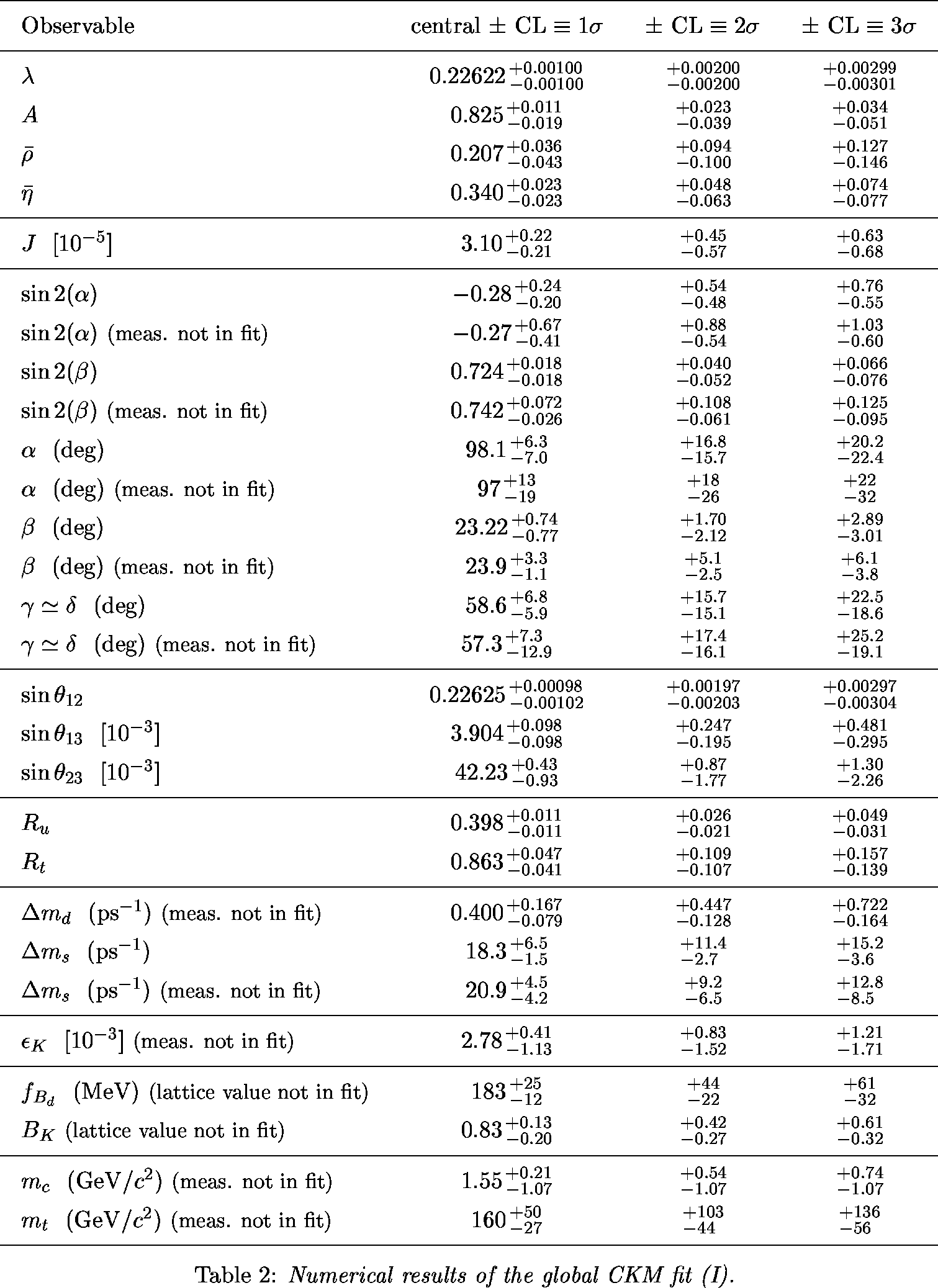ps pdf

ΔMs Results

 Comparison between CDF ΔMs measurement and CKM fit w/o ΔMs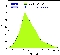eps gif |Vtd/Vts| from the global fit, the CDF ΔMs measurement and the ratio of the branching fractions (B→ρ0γ / B→K*0 γ) (average of BABAR and Belle)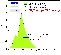eps gif

 α,β,γ convention: φ1,φ2,φ3 convention: Constraint in the (ρ-bar,η-bar) plane from the simultaneous use of the the B+→τ+ν branching fraction and Δmd.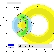eps gifeps gif

 α,β,γ convention: φ1,φ2,φ3 convention: Constraints in the (ρ-bar,η-bar) plane including (a.o.) the most recent α/Φ2- and γ/Φ3-related inputs in the global CKM fit.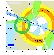eps gifeps gif

 Tree = |Vub / Vcb| + γ(DK) and γ(π - α(isospin) - β(charmonium)) α,β,γ convention: φ1,φ2,φ3 convention: Constraints from Tree and s to d transition in the (ρ-bar,η-bar) plane.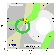eps gifeps gif Constraints from Tree and b to d transition in the (ρ-bar,η-bar) plane.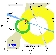eps gifeps gif Constraints from Tree and b to s transition in the (ρ-bar,η-bar) plane (sin2β: naive average from penguin modes).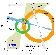eps gifeps gif Constraints from Tree and b to (d,s) transition in the (ρ-bar,η-bar) plane (sin2β: average between charmonium and penguin modes).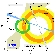eps gifeps gif

 α,β,γ convention: φ1,φ2,φ3 convention: Zoomed constraints in the (ρ-bar,η-bar) plane including the most recent α/Φ2- and γ/Φ3-related inputs in the global CKM fit.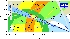eps gifeps gif Constraints in the (ρ-bar,η-bar) plane including only the angle measurements.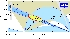eps gifeps gif Constraints from CP conserving quantities (|Vub / Vcb|, Δmd, (Δmd and Δms) and B+ →τ+ ν) in the (ρ-bar,η-bar) plane.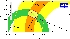eps gifeps gif Constraints from CP violating quantities (sin(2β), α, γ and εk) in the (ρ-bar,η-bar) plane.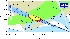eps gifeps gif Constraints from "Tree" quantities in the (ρ-bar,η-bar) plane (γ(DK) and α from the isospin analysis with the help of sin2β (charmonium), which gives another tree only γ measurement (the only assumption is that the ΔI=3/2 b-->d EW penguin amplitude is negligible)).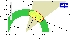eps gifeps gif Constraints from "Loop" quantities in the (ρ-bar,η-bar) plane.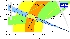eps gifeps gif

 Constraint on α/Φ2 from B→ππ.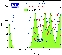eps gif Constraint on α/Φ2 from B→ρρ.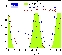eps gif Constraints on α/Φ2 from B→ππ (WA), ρπ(BABAR), ρρ(WA), compared to the prediction from the CKM fit (not including these measurements). α[combined] = 92.6 +10.7/–9.3 deg.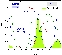eps gif The numerical results for these quantities are given in the summary paper

 Constraints on γ/Φ3 from world average D(*)K decays (GLW+ADS) and Dalitz analyses compared to the prediction from the global CKM fit (not including these measurements). γ[combined] = 62 +38/–24 deg.eps gif The numerical results for these quantities are given in the summary paper

 Constraints in the (rhobar,etabar) plane from the observables in B->pipi,Kpi,KKbar decays and using SU(3) flavor symmetry. In red the 2sigma contour of the standard CKM fit. (ICHEP 2006 data).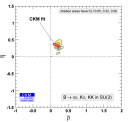eps gif The observables Rn vs S(Kspi0): indirect prediction from the SU(3) fit (without the corresponding experiment inputs) and the standard determination of CKM elements. In blue the 1sigma bands of the direct experimental measurements. (Moriond 2006 data).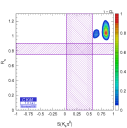eps gif The observables Rn vs S(Kspi0): indirect prediction from the SU(3) fit (without the corresponding experiment inputs) and the standard determination of CKM elements. In blue the 1sigma bands of the direct experimental measurements. (ICHEP 2006 data).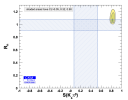eps gif

 New physics in B0- B0bar Mixing described model-independently by introducing two new parameters measuring the relative strength (rd2) and the relative phase between the B0- B0bar mixing matrix element containing contributions from SM as well as from NP contributions compared to SM contributions only.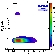eps gif